#5
14
11. 云栖社区>
2. 博客>
3. 正文

## 人工智能之机器学习算法体系汇总

Github开源机器学习系列文章及算法源码

# 1.人工智能之机器学习体系汇总

【直接上干货】此处梳理出面向人工智能的机器学习方法体系，主要体现机器学习方法和逻辑关系，理清机器学习脉络，后续文章会针对机器学习系列讲解算法原理和实战。抱着一颗严谨学习之心，有不当之处欢迎斧正。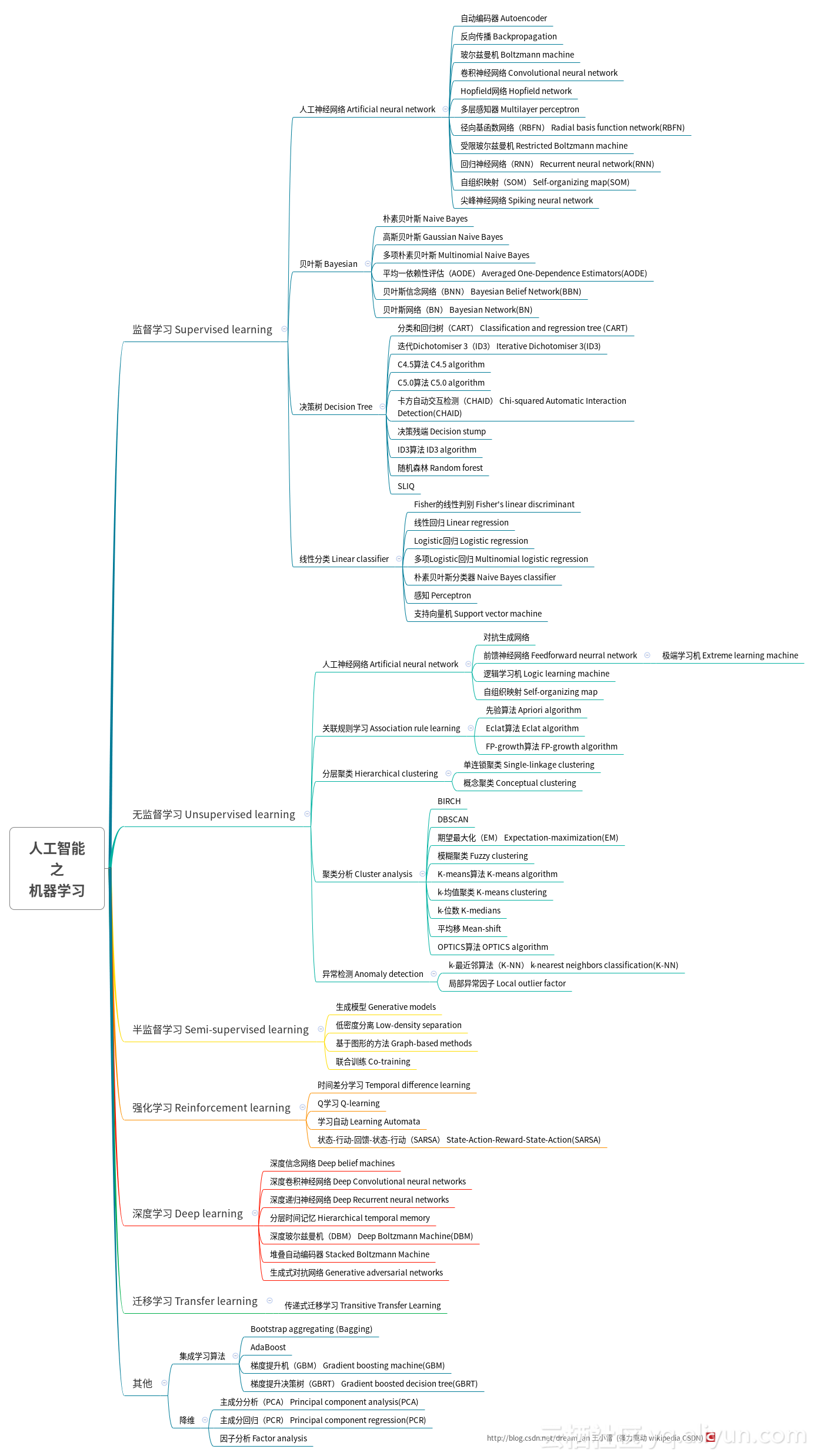• 监督学习 Supervised learning

• 人工神经网络 Artificial neural network

• 自动编码器 Autoencoder
• 反向传播 Backpropagation
• 玻尔兹曼机 Boltzmann machine
• 卷积神经网络 Convolutional neural network
• Hopfield网络 Hopfield network
• 多层感知器 Multilayer perceptron
• 径向基函数网络（RBFN） Radial basis function network(RBFN)
• 受限玻尔兹曼机 Restricted Boltzmann machine
• 回归神经网络（RNN） Recurrent neural network(RNN)
• 自组织映射（SOM） Self-organizing map(SOM)
• 尖峰神经网络 Spiking neural network
• 贝叶斯 Bayesian

• 朴素贝叶斯 Naive Bayes
• 高斯贝叶斯 Gaussian Naive Bayes
• 多项朴素贝叶斯 Multinomial Naive Bayes
• 平均一依赖性评估（AODE） Averaged One-Dependence Estimators(AODE)
• 贝叶斯信念网络（BNN） Bayesian Belief Network(BBN)
• 贝叶斯网络（BN） Bayesian Network(BN)
• 决策树 Decision Tree

• 分类和回归树（CART） Classification and regression tree (CART)
• 迭代Dichotomiser 3（ID3） Iterative Dichotomiser 3(ID3)
• C4.5算法 C4.5 algorithm
• C5.0算法 C5.0 algorithm
• 卡方自动交互检测（CHAID） Chi-squared Automatic Interaction Detection(CHAID)
• 决策残端 Decision stump
• ID3算法 ID3 algorithm
• 随机森林 Random forest
• SLIQ
• 线性分类 Linear classifier

• Fisher的线性判别 Fisher's linear discriminant
• 线性回归 Linear regression
• Logistic回归 Logistic regression
• 多项Logistic回归 Multinomial logistic regression
• 朴素贝叶斯分类器 Naive Bayes classifier
• 感知 Perceptron
• 支持向量机 Support vector machine
• 无监督学习 Unsupervised learning

• 人工神经网络 Artificial neural network

• 对抗生成网络
• 前馈神经网络 Feedforward neurral network

• 极端学习机 Extreme learning machine
• 逻辑学习机 Logic learning machine
• 自组织映射 Self-organizing map
• 关联规则学习 Association rule learning

• 先验算法 Apriori algorithm
• Eclat算法 Eclat algorithm
• FP-growth算法 FP-growth algorithm
• 分层聚类 Hierarchical clustering

• 概念聚类 Conceptual clustering
• 聚类分析 Cluster analysis

• BIRCH
• DBSCAN
• 期望最大化（EM） Expectation-maximization(EM)
• 模糊聚类 Fuzzy clustering
• K-means算法 K-means algorithm
• k-均值聚类 K-means clustering
• k-位数 K-medians
• 平均移 Mean-shift
• OPTICS算法 OPTICS algorithm
• 异常检测 Anomaly detection

• k-最近邻算法（K-NN） k-nearest neighbors classification(K-NN)
• 局部异常因子 Local outlier factor
• 半监督学习 Semi-supervised learning

• 生成模型 Generative models
• 低密度分离 Low-density separation
• 基于图形的方法 Graph-based methods
• 联合训练 Co-training
• 强化学习 Reinforcement learning

• 时间差分学习 Temporal difference learning
• Q学习 Q-learning
• 学习自动 Learning Automata
• 状态-行动-回馈-状态-行动（SARSA） State-Action-Reward-State-Action(SARSA)
• 深度学习 Deep learning

• 深度信念网络 Deep belief machines
• 深度卷积神经网络 Deep Convolutional neural networks
• 深度递归神经网络 Deep Recurrent neural networks
• 分层时间记忆 Hierarchical temporal memory
• 深度玻尔兹曼机（DBM） Deep Boltzmann Machine(DBM)
• 堆叠自动编码器 Stacked Boltzmann Machine
• 迁移学习 Transfer learning

• 传递式迁移学习 Transitive Transfer Learning
• 其他

• 集成学习算法

• Bootstrap aggregating (Bagging)
• 梯度提升决策树（GBRT） Gradient boosted decision tree(GBRT)
• 降维

• 主成分分析（PCA） Principal component analysis(PCA)
• 主成分回归（PCR） Principal component regression(PCR)
• 因子分析 Factor analysis

# 2.人工智能相关趋势分析

## 2.1.人工智能再次登上历史舞台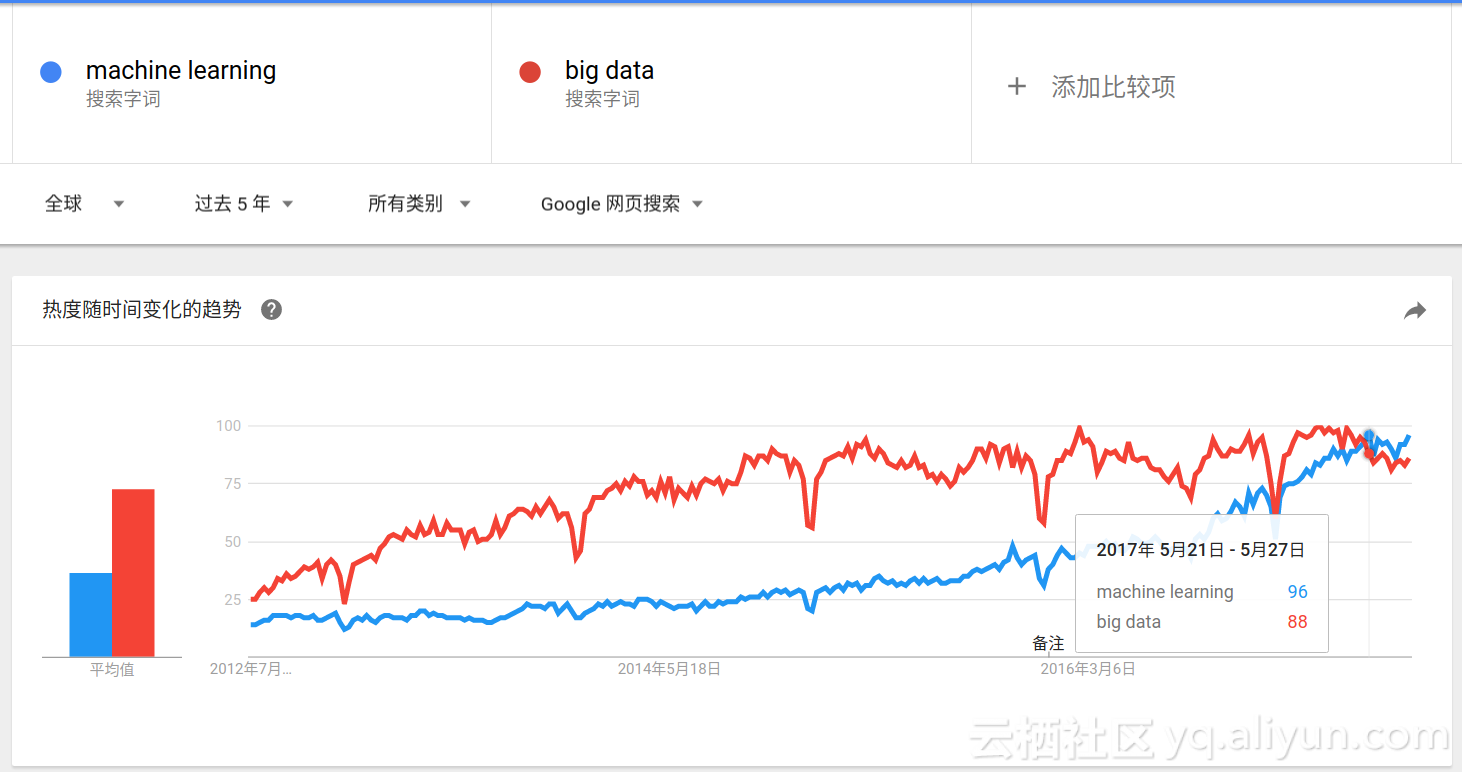[数据来自Goolge trends]

## 2.2.Python才是王道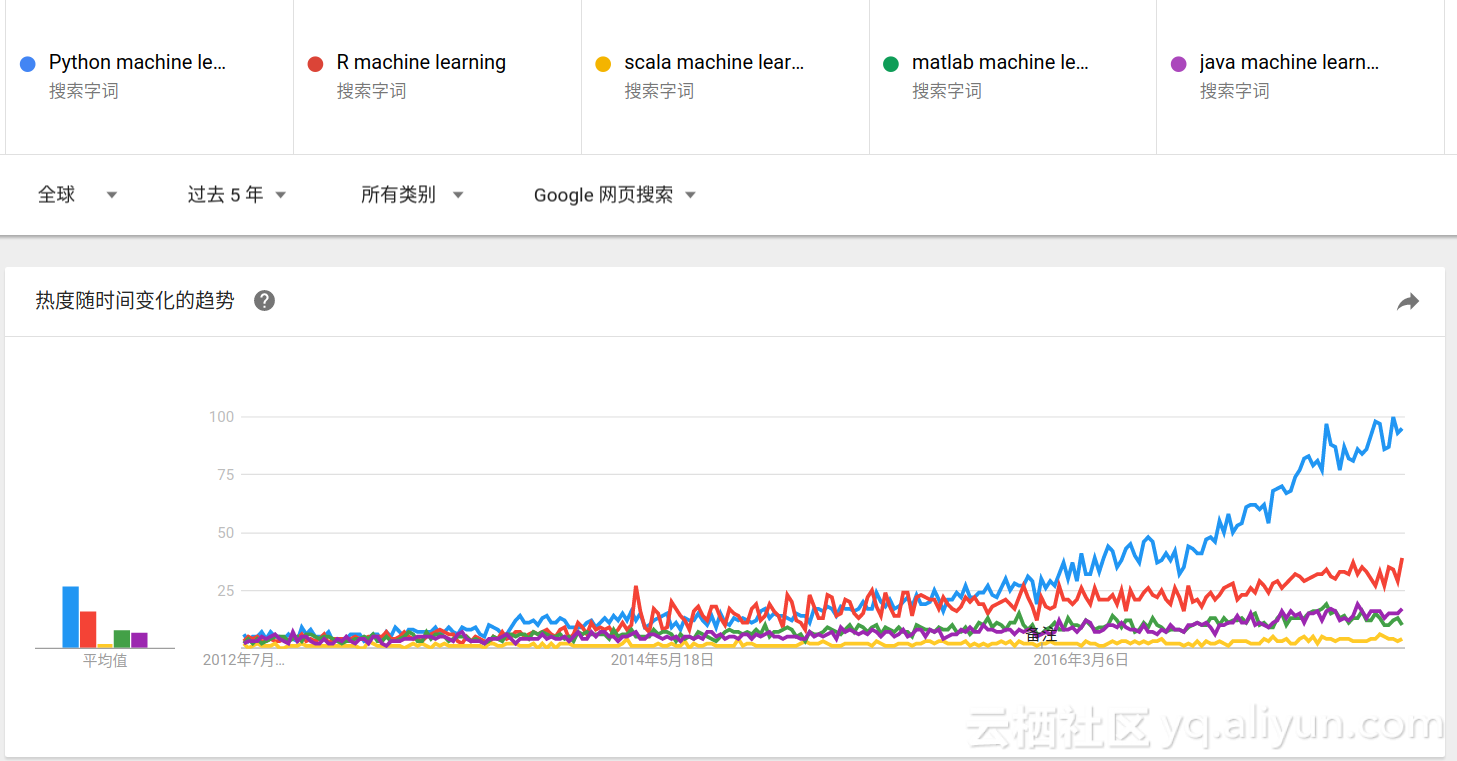## 2.3.深度学习趋势大热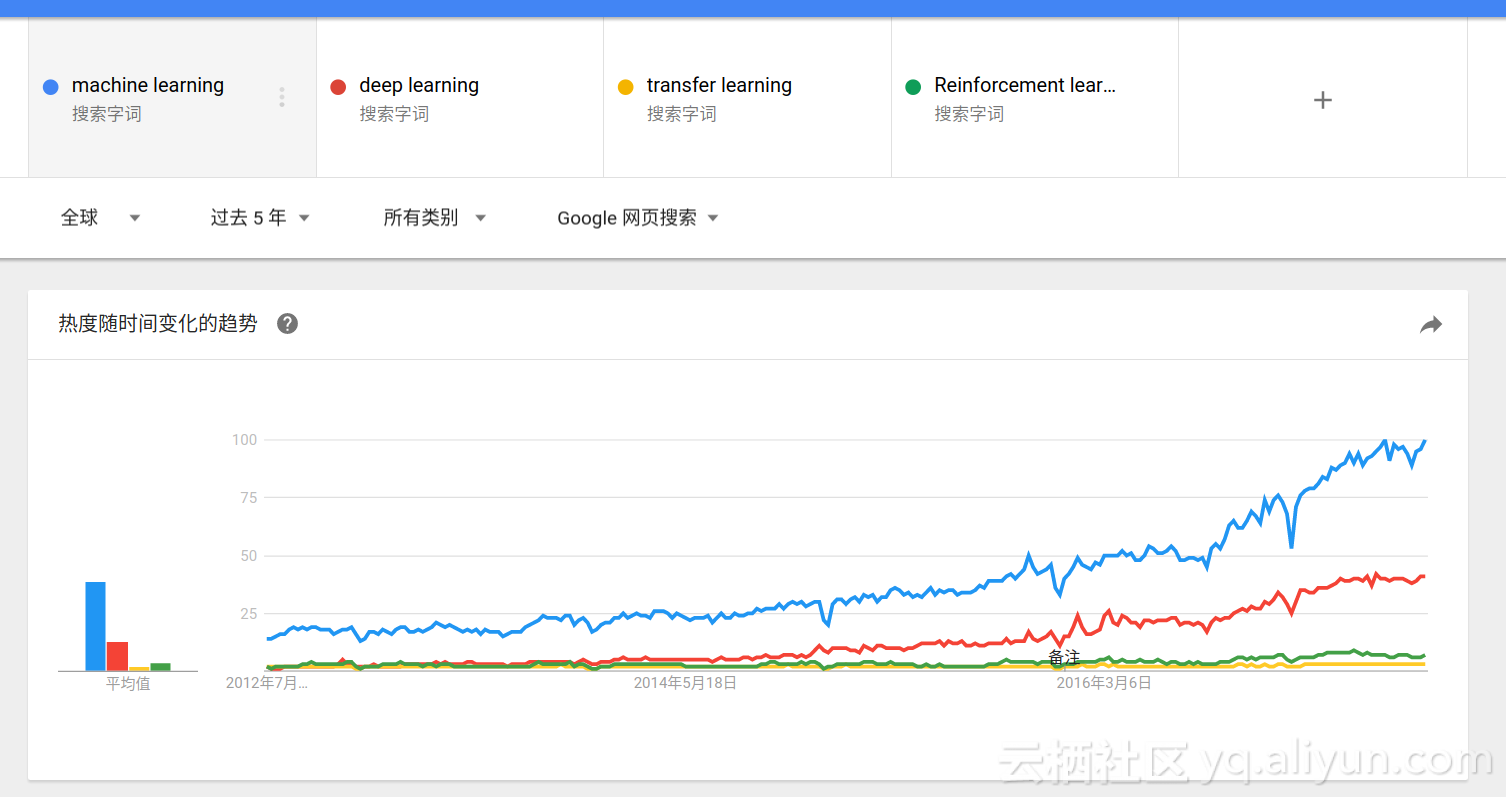## 2.4.中国更爱深度学习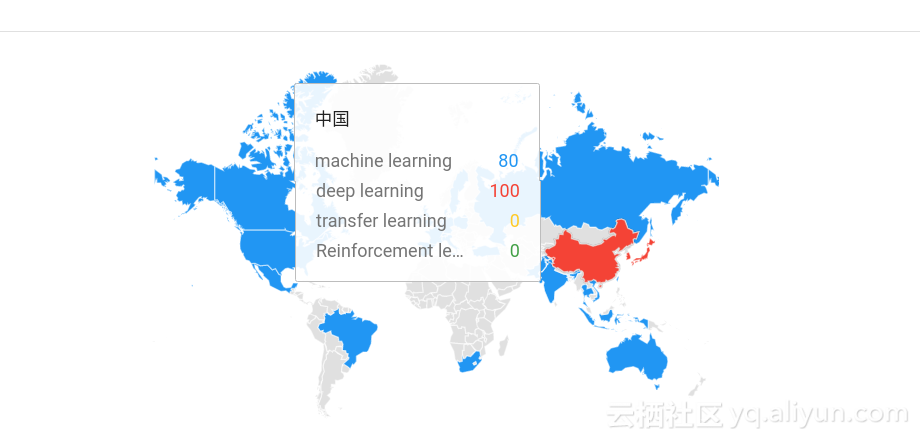# 3.结语

AI systems can’t model everything... AI needs to be robust to “unknown unknowns” [Thomas G.Dietterich ,2017CCAI]

“知之为知之，不知为不知，是知也”【出自《论语》】

+ 关注